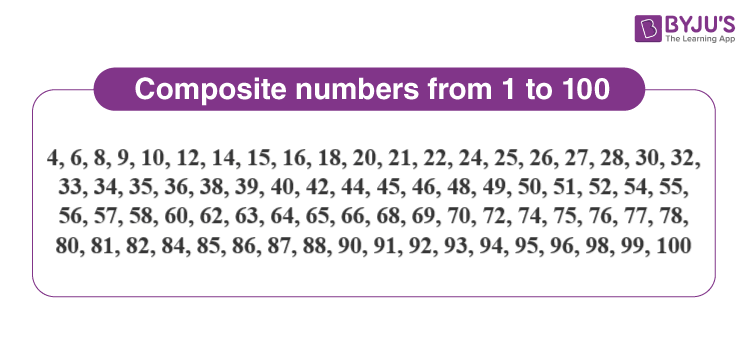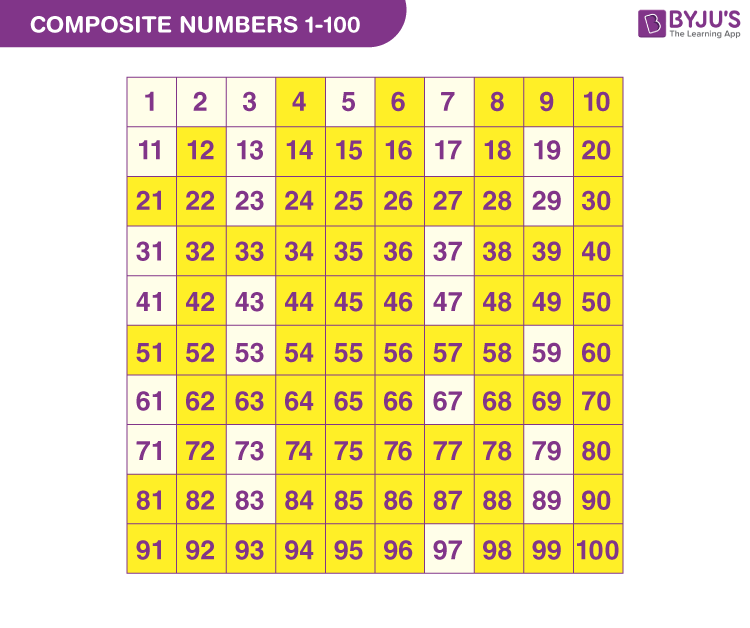# Composite Numbers

In Mathematics, composite numbers are numbers that have more than two factors. These numbers are also called composites. Composite numbers are just the opposite of prime numbers which have only two factors, i.e. 1 and the number itself. All the natural numbers which are not prime numbers are composite numbers as they can be divided by more than two numbers. For example, 6 is a composite number because it is divisible by 1, 2, 3 and even by 6. In this article, we will learn the definition of composite numbers, properties, smallest composite number, even and odd composite numbers, list of composite numbers, and difference between prime and composite numbers along with many solved examples in detail.

## What are Composite Numbers in Maths?

Definition 1: In Mathematics, composite numbers are numbers that have more than two factors.

Definition 2: The numbers which can be generated by multiplying the two smallest positive integers and contain at least one divisor other than the number ‘1’ and itself are known as composite numbers. These numbers always have more than two factors.

Fact: Any even number which is greater than 2 is a composite number.

### Is 0 a Composite Number?

Zero (0) is considered as neither prime nor a composite number because it does not have any factors.

## Composite Numbers Examples

The examples of composite numbers are 6, 14, 25, 30, 52, etc, such that:

 Composite numbers Factors 6 1, 2, 3, 6 14 1, 2, 7, 14 25 1, 5, 25 30 1, 2, 3, 5, 6, 10, 15, 30 52 1, 2, 4, 13, 26, 52

In all the above examples, we can see the composite numbers have more than two factors. There are a number of composite numbers we can list out of a set of natural numbers from 1 to 1000 or more. Let us see the list of composite numbers in the next section.

## Properties of Composite Numbers

The properties of composite numbers are easy to remember.

• Composite numbers have more than two factors
• Composite numbers are evenly divisible by their factors
• Each composite number is a factor of itself
• The smallest composite number is 4
• Each composite number will include at least two prime numbers as its factors (Eg. 10 = 2 x 5, where 2 and 5 are prime numbers)
• Composite numbers are divisible by other composite numbers also

## List of Composite Numbers

Here is the list of composite numbers from 1 to 100 in Maths. Students can keep a note of this and also try to write the numbers beyond 100 for practice, such composites from 1 to 200 or till 500.## Composite Numbers Chart## Composite Numbers 1 to 200

The positive integers having more than two factors are composite numbers. The list of Composite numbers from 1 to 200 are given below in the table.

 4, 6, 8, 9, 10, 12, 14, 15, 16, 18, 20, 21, 22, 24, 25, 26, 27, 28, 30, 32, 33, 34, 35, 36, 38, 39, 40, 42, 44, 45, 46, 48, 49, 50, 51, 52, 54, 55, 56, 57, 58, 60, 62, 63, 64, 65, 66, 68, 69, 70, 72, 74, 75, 76, 77, 78, 80, 81, 82, 84, 85, 86, 87, 88, 90, 91, 92, 93, 94, 95, 96, 98, 99, 100, 102, 104, 105, 106, 108, 110, 111, 112, 114, 115, 116, 117, 118, 119, 120, 121, 122, 123, 124, 125, 126, 128, 129, 130, 132, 133, 134, 135, 136, 138, 140, 141, 142, 143, 144, 145, 146, 147, 148, 150,  152, 154, 155, 156, 157, 158, 159, 160, 162, 164, 165, 166, 168, 170, 172, 174, 175, 176, 177, 178, 180, 182, 184, 185, 186, 187, 188, 189, 190, 192, 194, 195, 196, and 198.

## How to Find the Composite Number?

The procedures to find whether a given number is prime or composite:

• Find all the factors of the positive integer
• A number is said to be prime if it has only two factors, 1 and itself
• If the number has more than two factors, then it is a composite
 Example: Find if 14 is a composite number. Let us find the factors of 14. 14÷1 = 14 14÷2 = 7 14÷7 = 2 14÷14 = 1 As we can see, the factors of 14 are 1,2,7 and 14, so it is a composite number.

## Types of Composite Numbers

There are two main types of composite numbers in Maths which are:

• Odd Composite Numbers or Composite Odd Numbers
• Even Composite Numbers or Composite Even Numbers

### Odd Composite Numbers

All the odd integers which are not prime are odd composite numbers. Examples of composite odd numbers are 9, 15, 21, 25, 27, 31, etc.

### Even Composite Numbers

All the even integers which are not prime are even composite numbers. Examples of even composite numbers are 4, 6, 8, 10, 12, 14, 16, etc.

## Smallest Composite Number

4 is the smallest composite number.

Why?

1 is not a composite number because the sole divisor of 1 is 1. The positive integers 2 and 3 are prime numbers because it can be divided by only two factors, one and itself. Hence 2 and 3 are not composite.

But in the case of number 4, we have more than two factors. The divisors of 4 are 1,2,4. So this number satisfies the condition of a composite number as mentioned above. After 4, 6 is the next composite positive integer, which has factors 1, 2, 3 and 6.

Hence, 4 is the smallest composite number (Proved).

 Important Notes on Composite numbers Smallest Composite Number = 4 Smallest Prime Number = 2 Smallest Odd Composite Number = 9 Two-digit Smallest Composite Number = 10

## Difference Between Prime and Composite Numbers

The difference between the prime numbers and the composite numbers in Maths are listed below:

Prime Numbers Composite Numbers
It can only be divided by 1 and itself, thus have only two factors. It has more than two factors (1 and itself).
It can only be written as a product of two numbers It can be written as the product of two or more numbers
Example: 5 has factors are 1 and 5 Example: 4 has factors are 1, 2 and 4

## Prime Factorization of Composite Numbers

The list of composite numerals from 1 to 50 are given here with their prime factorization. You can see here how the composites are factorized in prime numbers. Check the below table to understand better. With the help of this table, you can also find composites beyond 50 with their prime factorization.

 Composite Numbers Prime Factorization 4 2 × 2 6 2 × 3 8 2 × 2 × 2 9 3 × 3 10 2 × 5 12 2 × 2 × 3 14 2 × 7 15 3 × 5 16 2 × 2 × 2 × 2 18 2 × 3 × 3 20 2 × 2 × 5 21 3 × 7 22 2 × 11 24 2 × 2 × 2 × 3 25 5 × 5 26 2 × 13 27 3 × 3 × 3 28 2 × 2 × 7 30 2 × 3 × 5 32 2 × 2 × 2 × 2 × 2 33 3 × 11 34 2 × 17 35 5 × 7 36 2 × 2 × 3 × 3 38 2 × 19 39 3  × 13 40 2 × 2 × 2 × 5 42 2 × 3 × 7 44 2 × 2 × 11 45 3 × 3 × 5 46 2 × 23 48 2 × 2 × 2 × 2 × 3 49 7 × 7 50 2 × 5 × 5

## Video Lesson on Numbers## Solved Problems on Composite Numbers

Example 1:

Find if 328 is a composite number.

Solution:

The factors of 328 are 1, 2, 4, 8, 41, 82, 164, 328.

Therefore, 328 is a composite number.

Example 2:

What is the prime factorization of 60?

Solution:

The prime factorization of 60 is:

60 = 2 × 2 × 3 × 5.

Example 3:

List out the composite numbers from the given set of numbers.

2, 4, 9, 11, 21, 31, 44, 53, 67, 88, 101, 108.

Solution:

The composite numbers are: 4, 9, 21, 44, 88, 108.

Example 4:

Find the product of first 5 composite numbers.

Solution:

The first 5 composite numbers are 4, 6, 8, 9, 10.

Hence, the product of first 5 composite numbers = 4 × 6 × 8 × 9 × 10

= 17280

Therefore, the product of first five composite numbers is 17280.

## Practice Questions (Worksheet)

1. List the composite numbers between 20 and 30.
2. Find if 85 is a composite number.
3. What are the prime factors of 99?
4. What is the sum of factors of composite number 18?
5. Find the composite numbers among the following set of numbers in the given table.
 7 18 33 11 6 13 90 21 98 5 63 19 61 52 2 81

## Frequently Asked Questions on Composite Numbers

Q1

### What is a composite number?

A composite number is a natural number or a positive integer which has more than two factors. For example, 15 has factors 1, 3, 5 and 15, hence it is a composite number.
Q2

### Is 2 a composite number?

No, 2 is not a composite number because it has only two factors, i.e. 1 and 2. Hence, it is a prime number.
Q3

### Is 9 a composite number?

Yes, 9 is a composite number because it has more than two factors, such as 1, 3 and 9.
Q4

### What are the composite numbers from 1 to 100?

The list of composite numbers from 1 to 100 are:
4, 6, 8, 9, 10, 12, 14, 15, 16, 18, 20, 21, 22, 24, 25, 26, 27, 28, 30, 32, 33, 34, 35, 36, 38, 39, 40, 42, 44, 45, 46, 48, 49, 50, 51, 52, 54, 55, 56, 57, 58, 60, 62, 63, 64, 65, 66, 68, 69, 70, 72, 74, 75, 76, 77, 78, 80, 81,82, 84, 85, 86, 87, 88, 90, 91, 92, 93, 94, 95, 96, 98, 99, 100.
Q5

### Is 19 prime or composite?

19 is a prime number because it has only two factors, i.e. 1 and 19.
Q6

### Is 1 a composite number?

No, because 1 does not have more than two factors instead it has only 1 factor. Hence, it is neither prime nor composite.
Q7

### How many composite numbers are there between 1 and 10?

There are four composite numbers between 1 and 10. They are 4,6,8 and 9.
Q8

### Is 49 a composite number?

Yes, 49 is a composite number, because it contains more than 2 factors. The factors of 49 are 1, 7 and 49.

Test your knowledge on Composite Numbers